Computers, Materials & ContinuaDOI:10.32604/cmc.2022.020103Article

A Quantum Algorithm for Evaluating the Hamming Distance

1Department of Artificial Intelligence, Hurghada Faculty of Computers and Artificial Intelligence, South Valley University, Egypt
2Faculty of Engineering, King Salman International University, South Sinai, Egypt
3Department of Mathematics and Computer Science, Faculty of Science, Beni-Suef University, Beni-Suef, Egypt
4Department of Machine Learning and Information Retrieval, Faculty of Artificial Intelligence, Kafrelsheikh University, Egypt
5Mechanical Department, Faculty of Technology and Education, Suez University, Suez, Egypt
6Faculty of Technological Industries, King Salman International University, South Sinai, Egypt
7Faculty of Engineering at Mataria, Helwan University, Cairo, Egypt
8Department of Mathematics, Faculty of Sciences, Sohag University, 82524 Sohag, Egypt
*Corresponding Author: Mohammed Zidan. Email: comsi2014@gmail.com
Received: 09 May 2021; Accepted: 29 July 2021

Abstract: We present a novel quantum algorithm to evaluate the hamming distance between two unknown oracles via measuring the degree of entanglement between two ancillary qubits. In particular, we use the power of the entanglement degree based quantum computing model that preserves at most the locality of interactions within the quantum model structure. This model uses one of two techniques to retrieve the solution of a quantum computing problem at hand. In the first technique, the solution of the problem is obtained based on whether there is an entanglement between the two ancillary qubits or not. In the second, the solution of the quantum computing problem is obtained as a function in the concurrence value, and the number of states that can be generated from the Boolean variables. The proposed algorithm receives two oracles, each oracle represents an unknown Boolean function, then it measures the hamming distance between these two oracles. The hamming distance is evaluated based on the second technique. It is shown that the proposed algorithm provides exponential speedup compared with the classical counterpart for Boolean functions that have large numbers of Boolean variables. The proposed algorithm is explained via a case study. Finally, employing recently developed experimental techniques, the proposed algorithm has been verified using IBM's quantum computer simulator.

Keywords: Quantum computing; quantum algorithm; quantum circuit

1  Introduction

Quantum algorithms have enormous technological and recent progress to solve the problem that needs high-performance computing on traditional computers . Nowadays, quantum technologies stand at the crossroads between many areas of study, such as quantum information, combinatorics, computational complexity, and statistical mechanics .

Boolean functions play a critical role in cryptography, particularly in the design of symmetric key algorithms. Analyzing these functions can be done using many techniques, such as spectral techniques. The Hamming distance H(f,g) is the natural distance between two binary strings. In 2014, De et al.  showed a quantum algorithm based on the Chow parameters problem of any n- bit linear threshold function f given that a high prodigality in the Hamming distance which runs in time O(n2).(1ϵ)O(log2(1ϵ)).

Xie et al.  have calculated H(f,g) with n inputs that require O(1) with probability at least 8/π2 in some special cases by taking advantage of Walsh transform. Moreover, they proposed an optimal algorithm which requires θ(2n) the exact query complexity with accuracy O(N.ϵ2t+1) classically. Other researchers proposed algorithms based on development of two Hamming distance using unlike types of inner products like gnomic quantum classifier . In the context of quantum communication complexity, Doriguello et al.  proposed an efficient quantum communication protocol to approximately measure the Hamming distance between two n bit strings in the SMP model with some relative error ϵ. Their protocol uses O(lognϵ5)qubits of communication.

Recently, it was proposed that the degree of entanglement can be used efficiently to develop new quantum computing model . A generalized version of the Deutsch-Jozsa problem was solved based on this quantum computing model . Also, this model was used to propose quantum machine leaning algorithms based on this quantum computing model to perform competitive learning quantum mechanically [12,13]. Moreover, based on symmetric matrices in quantum stabilizer codes, a construction of binary and non-binary quantum stabilizer codes is presented by [14,15]. In this paper, a novel quantum algorithm computes the Hamming distance Ham(f, g) between two unknown Boolean functions. Concretely, it is proposed based on adding four ancilla qubits, and two extra CNOT gates in addition to the degree of entanglement-based quantum computing model . The proposed algorithm retrieves Ham(f, g) between two given oracles Uf and Ug. Then, the complexity of the proposed algorithm is compared with the classical algorithm. Finally, the proposed algorithm is verified using IBM's quantum computer simulator.

The next part of this paper is organized, as follows: In Section 2, the methodology that is used to propose the algorithm is explained. Section 3 shows the problem statement, the proposed algorithm and analysis of the performance of the proposed algorithm via a case study. Also, the complexity of the proposed algorithm compared with classical algorithm is investigated in Section 3. Verification of the proposed algorithm on IBM's quantum computer simulator, and results discussion is performed in Section 4. Finally, main conclusions are discussed in Section 5.

2  Methodology

Recently, quantum computing model based on the degree of entanglement has been proposed . This model utilizes concurrence measure to find the solution of some quantum problems based on the degree of entanglement C between two auxiliary qubits in a quantum system of n + 2, where n ≥ 0 [7,1113]. In this model, the concurrence value C is estimated quantum mechanically via the operator Mz. Therefore, the solution of the quantum computing problems is obtained based on C. Moreover, it was proved that the operator Mz has the potential to distinguish between non-orthogonal states in the form a0|0+b0|1 by quantifying the degree of entanglement between two qubits . Suppose we have an arbitrary qubit in the state presented in Eq. (1) and demonstrated in Fig. 1.

|f(x)=a0 |0+b0 |1,  |a0|2+|b0|2=1.(1)Figure 1: The circuit model of the operator MZ that creates entanglement between the qubits |f(x)〉 and |s〉. Then, it measures the concurrence value quantum mechanically via C=2(P0011+P1100)

Mz operator receives two decoupled replicas of two inputs. The first input is the qubit

|f(x) which has a state described by Eq. (1). The second input is a qubit |s which is initialized in the state |0 while the operator Mz applies two operations.

In the first operation, the operator Mz creates entanglement between each replica of the two qubits |f(x)〉 and |s〉 individually and simultaneously. Hence, the state of each replica is defined by the state presented in Eq. (2).

|f(x)s=a0 |00+b0 |11.(2)

This operation is achieved by applying CNOT on each replica of the two qubits |f(x) and |s〉 individually and simultaneously, where the control and the target qubits are |f(x) and |s〉, respectively. The concurrence value C for the state described by Eq. (2) is defined as in Eq. (3) :

C=2|a0b0|.(3)

In the second operation, the operator Mz measures C between the two qubits |f(x)s〉 by measuring the following two replica |f(x)s|f(x)s. Then, the concurrence value C between the two qubits |f(x) and |s〉 is quantified on a quantum chip as shown in Eq. (4).

C=2(P0011+P1100),(4)

where P0011, P1100 are the probabilities of the states |0011, |1100〉, respectively. Therefore, the first technique of this computing model  finds the solution of the problem at hand based on the degree of entanglement C.

3  The Proposed Algorithm

In this section, we investigate the proposed algorithm for measuring the hamming distance between two functions including the problem statement, proposed algorithm, and performance analysis of the proposed algorithm by presenting a case study as in the following subsections.

3.1 Problem Statement

The Hamming distance between two functions f and g, each acting on n-variable provided via two black-boxes, is defined according to the following definition:

•   Definition: The Hamming distance Ham(f, g) between two Boolean functions f(x) and g(x) of n− Boolean variables is defined as:-

Ham(f(x),g(x))=|{x{0,1}n:f(x)g(x)}|

The abstract problem of this paper can be defined, as follows:

•   Given: Given two oracles represent two unknown Boolean functions such that

Uf:{0,1}n{0,1},and Ug:{0,1}n{0,1}.

•   Goal: Retrieve the Hamming distance Hamϵ(f,g) between f(x) and g(x) such that:

(1ϵ)Ham(f,g)Hamϵ(f,g)(1+ϵ)Ham(f,g).

3.2 The Proposed Algorithm

Now, the proposed algorithm that solves the above problem can be described, as follows:

(1) Step 1: Initialize the quantum register |ψ of size n qubits, two disentangled qubits namely: |yf,|yg, and a two-qubit register |ϕ. All of them are initialized in the vacuum state, |0, as follows:

|ζ0=|ψn|yf,yg,ϕ=|0n|0,0,00.

(2) Step 2: Apply the Hadamard gates to the first n qubits.

|ζ1=(HnI4)|ζ0=12nx=02n1|x|0,0,00,

(3) Step 3: Apply the oracle Uf and on the first n qubits and the qubit |yf, then the oracle Ug and on the first n qubits and the qubit |yg:

Uf|x,yf=|x|yff(x)=|x|0f(x)=|x|f(x), Ug|x,yg=|x|ygg(x)=|x|0g(x)=|x|g(x). So, the state of the system is as follows: |ζ2=12nx=02n1|x|f(x),g(x),00.

(4) Step 4: Apply the CNOT gate twice, as follows:

|ζ3=CNOTyfϕ1CNOTygϕ1|ζ2.

(5) Step 5: Repeat steps 1, 2, 3 and 4 to get a novel decoupled copy of the system |ζ3.

(6) Step 6: Apply Mz between on the two replica of the two qubits |ϕ1 and |ϕ2,

If P0000>P1111, then Ham(f(x),g(x)) as in Eq. (5).

Ham(f(x),g(x))=N2(11C2).(5)

If P1111P0000, then Ham(f(x),g(x)) as in Eq. (6).

Ham(f(x),g(x))=N2(1+1C2),(6)

where C is determined as in Eq. (4).

3.3 Performance Analysis of the Proposed Algorithm: Case Study

Here, we analyze the performance of the proposed algorithm via a case study. Assume we have the two Boolean functions f(x) and g(x), such that n = 2, and corresponding results are defined as in Eq. (7).

f(00)=0,f(01)=0,f(10)=1,andf(11)=1,

g(00)=0,g(01)=1,g(10)=0,andg(11)=1.(7)

To determine the Hamming distance between f(x) and g(x), the steps of the proposed algorithm act, as follows: In Step1, because the number of Boolean variables is 2, so the size of the register |ψ is n = 2 qubits. Consequently, the whole quantum system is in the following state:

|ζ0=|ψn=2|yf,yg,ϕ=|ψ2|yf,yg,ϕ=|00,0,0,00.

In Step 2, the proposed algorithm applies 2 Hadamard gates on the first two qubits of the system |ζ0, so the state of the system is transformed to the following state:

|ζ1=(H2I4|ζ0=14(|00,0,0,00+|01,0,0,00+|10,0,0,00+|11,0,0,00).

This step generates the whole domain of the two Boolean functions f(x) and g(x).

In Step 3, the proposed algorithm applies the oracle Uf on the first n qubits and the qubit |yf, and applies the oracle Ug and on the first n qubits and the qubit |yg at the same time. Therefore, the state of the system after applying the Oracle Uf is as in Eq. (8).

|ζ2=|x0x1,yff(x0x1),0,00)=12x=03|x0x1,|f(x),0,00 =14[|00,0,0,00+|01,0,0,00+|10,1,0,00+|11,0,0,00].(8)

Similarly, the state of the system after applying the Oracle Ug is as in Eq. (9).

|ζ2=|x0x1,f(x), ygg(x0x1),00)=12x=03|x0x1,|f(x),g(x),00 =14[|00,0,0,00+|01,0,1,00+|10,1,0,00+|11,1,1,00].(9)

In Step 4, the proposed algorithm applies 2 CNOT gates simultaneously as follows:

|ζ3=CNOTyfϕ1CNOTygϕ1,|ζ2.

By applying the CNOTygϕ1gate, the control qubit |yg and the target qubit |ϕ1. Therefore the state of the entire system is evolved as shown in Eq. (10).

|ζ3=14[|00,0,0,00+|01,0,1,10+|10,1,0,00+|11,1,1,10](10)

At the same time, by applying the CNOTyfϕ1 gate between the control qubit |yf and the target qubit |ϕ1. Then, the state of the system is described as in Eq. (11).

|ζ3=14[|00,0,0,00+|01,0,1,10+|10,1,0,10+|11,1,1,00](11)

Now, it is clear from Eq. (11) that step 4 evolves the state of the qubit |ϕ1  to be in state |1 only if the two functions f(xi)g(xi). On other hand, the state of the qubit |ϕ1 is the state |0 only if f(xi)=g(xi) for xi. In Step 5, the steps 1–4 are repeated to construct another decoupled replica of the system |ζ3, because Mz operator works on two decoupled copies of the register |ϕ to quantify the degree of entanglement between the two qubits: |ϕ1 and |ϕ2 (see Section 2). In Step 6, the proposed algorithm applies the two operations of the operator Mz between the two qubits |ϕ1 and |ϕ2, for each replica, in the system defined by Eq. (11). In the first operation, the operator Mz applies the CNOTφ1φ2 gate on each replica, where the |ϕ1 is the control qubit and |ϕ2 is the target qubit. In the general case, n Boolean variables, the state of each replica after applying the first operation of the operator Mz is described as in Eq. (12):

|ζ4=(In+2CONT|ζ3=t12n(1t1x={x|f(x)=g(x) }yfyg={00,11}|x|yfyfg)|00+t22n(1t2x={x|f(x)g(x) }yfyg={01,10}|x|yfyfg)|11(12)

Let,

|β1=x={x|f(x)=g(x)}yfyg={00,11}|x|yfyfg, |β2=x={x|f(x)=g(x)}yfyg{01,10}|x|yfyfga0=t12n, and b0=t22n.(13)

Thus, Eqs. (12)(13), the state system is summarized in Eq. (14).

|ξ4=a0|β1|00+b0|β2|11.(14)

According to Eq. (14), the first operation of the operator Mz applies the CNOTφ1φ2 to entangle the two qubits: |ϕ1 and |ϕ2, where the degree of entanglement in between depends on the value of the hamming distance among the two-function f(x) and g(x) (Ham(f(x), g(x)) > 0). Conversely, the operator Mz maintains the two qubits: |ϕ1 and |ϕ2 separable only if the hamming distance among the two-function f(x) and g(x) (Ham(f(x), g(x)) = 0).

Now, for this case study at hands, n = 2 and |ζ3 is defined by Eq. (11). The state of each replica after applying the first operation of the operator Mz is described according to Eq. (11) and Eqs. (12)(14), as in Eq. (15).

|ζ4=14[|00,0,0,00+|01,0,1,11+|10,1,0,11+|11,1,1,00].=t12n(1t1x={x|f(x)=g(x) }yfyg={00,11}|x|yfyg)|00+t22n(1t2x={x|f(x)g(x) }yfyg={01,10}|x|yfyg)|11=24(12x={00,11 }yfyg={00,11}|x|yfyg)+24(12x={01,10 }yfyg={01,10}|x|yfyg)|11=24 |β1 |00+24 |β2 |11.(15)

Consequently, the state of the system which consists of the two replicas, after applying the first operation of the operator Mz, is in Eq. (16).

|ξ=|ξ4|ξ4=24|β12|0000+24|β1|β2|0011  + 24|β2|β1|1100+24|β12|1111(16)

In the second operation of the operator Mz, the last four qubits in Eq. (16), are measured and the probabilities P0000, P1100, P0011, and P1111 are estimated. Then, the degree of entanglement C among the two qubits of the register |ϕ is quantified using Eq. (4) (see Section 2). In this case study, the concurrence value C according to the Eqs. (4) and (16) is C=2(P0011+P1100) . Therefore, C=2(416+416)=1. It is obvious from Eq. (16), that P0000 = P1111 after measuring operation. So, according to step 6 in the proposed algorithm (see Section (3.2)), the Hamming distance between the Boolean functions f(x) and g(x) is investigated as follows

Ham(f(x),g(x))=N2(1+1c2)=42(1+11)=2.

which can be verified from Eq. (7).

3.4 Complexity

Here, the complexity of the proposed algorithm is investigated. In quantum computing, the complexity of algorithms is calculated from the number of the oracle calls. It is evident from Eqs. (5)–(6) that the Hamming distance between the oracles Uf, and Ug depends on the value of the concurrence value C. This value can be determined based on the probabilities of states |0011, and |1100, for the last four qubits, |ϕ |ϕ, in Eq. (16). The proposed algorithm computes the probabilities of these states based on recall the oracles: Uf, and Ug via 2M times, where M is the number of measurements. Therefore, C is quantified by Eq. (4) with max error ϵ=12M. In IBM's real quantum computer , the number of measurements is M = 8192. Hence, the proposed algorithm needs to recall the oracles: Uf, and Ug with 2 M = 2(8192) = 214 times on this real quantum computer. Fig. 2 shows a comparison between the complexity of the proposed algorithm and that of classical algorithm. The elaboration of this Figure can be shown as follows: (i) It is evident from Fig. 2 that if the number of variables in the two oracles Uf, and Ug is 1n12, then time-cost of the proposed quantum algorithm is higher than the classical algorithm. (ii) If the number of variables in the two oracles Uf, and Ug is n = 13, then complexity of the proposed quantum algorithm and the classical algorithm is the same. (iii) It is evident from Fig. 2 that if the number of variables in the two oracles Uf, and Ug is n > 13, then the speed of the proposed algorithm increases dramatically compared with classical algorithm. The speed up of the proposed algorithm seems to be exponential when n > 18 if the max target error is 0.01.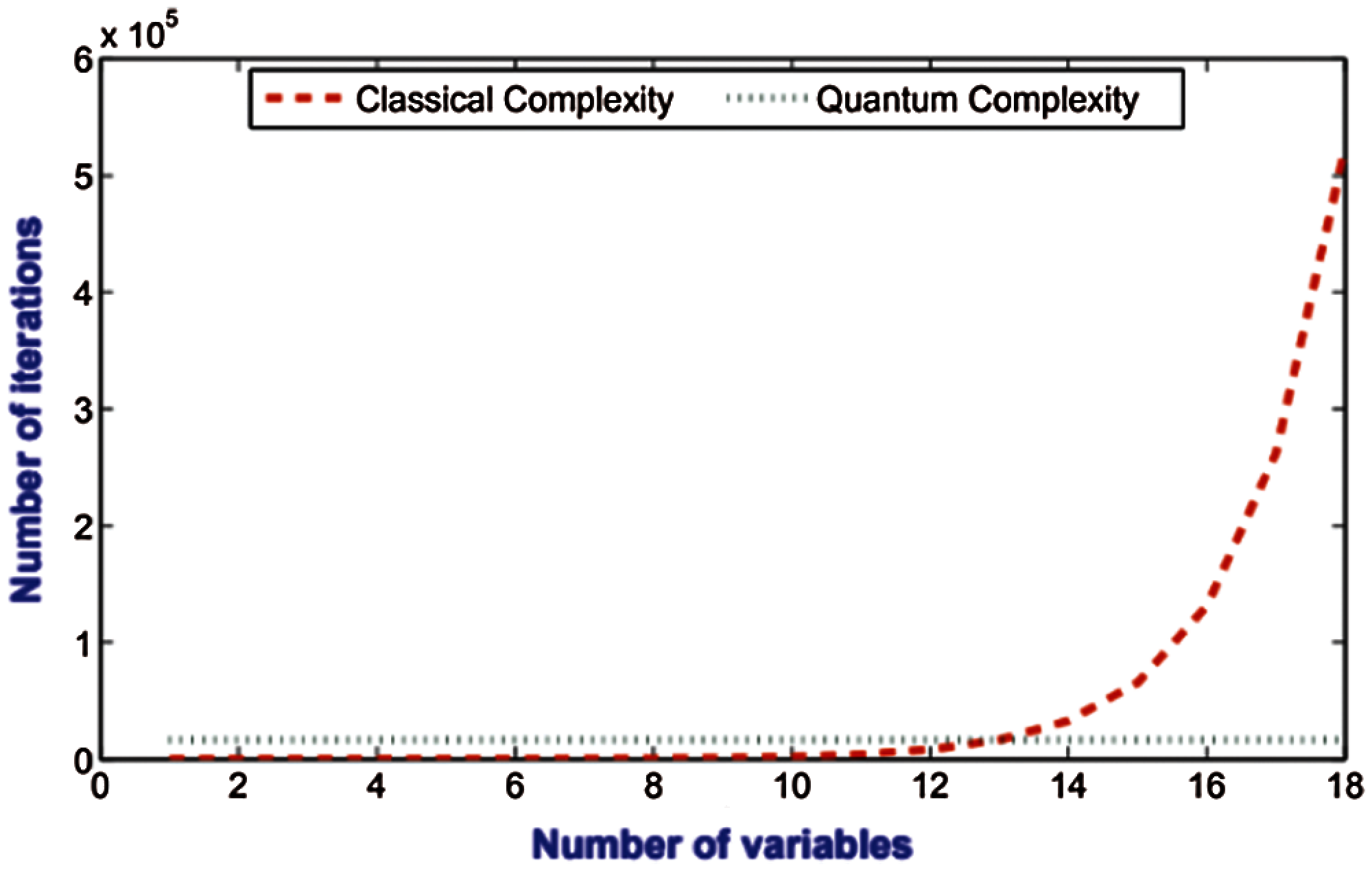Figure 2: Comparison between the quantum complexity and the classical complexity

4  Experimental Verification of the Proposed Algorithm

4.1 Experimental Setup

To verify the proposed algorithm practically, we will conduct some experiments for measuring the hamming distance between two oracles on IBM's quantum computer simulator called Qiskit Aer . Here, for verification purpose, it is assumed that these oracles are well known for the examiner but unknown for each body else. In the conducted experiments, the oracles, Uf and Ug, represent a Boolean function of two Boolean variables f : {0, 1}2 → {0, 1} and g: {0, 1}2 → {0, 1}, respectively. Thus, the possible hamming distances between these two oracles are Ham(f(x), g(x)) = {0, 1, 2, 3, 4}. Therefore, five experiments are conducted. In the first simulation experiment, the two oracles Uf and Ug are implemented via the Boolean functions f(x0, x1) = 1 and g(x0, x1) = 1, respectively. The quantum circuits of these oracles are shown in Fig. 3. In the second simulation experiment, the two oracles Uf and Ug are implemented via the Boolean functions f(x0, x1) = x1, and g(x0, x1) = x0x1, respectively. The quantum circuits of these oracles are shown in Fig. 4. In the third simulation experiment, the two oracles Uf and Ug are implemented via the Boolean functions f(x0, x1) = x0x1, and g(x0, x1) = x0x1x1, respectively. The quantum circuits of these oracles are shown in Fig. 5. In the fourth simulation experiment, the two oracles Uf and Ug are implemented via the Boolean functions f(x0, x1) = x0 ⊕ x1 and g(x0, x1) = x0x1, respectively. The quantum circuits of these oracles are shown in Fig. 6. In the last simulation experiment, the two oracles Uf and Ug are implemented via the Boolean functions f(x0, x1) = 1, and g(x0, x1) = 0, respectively. The quantum circuits of these oracles are shown in Fig. 7.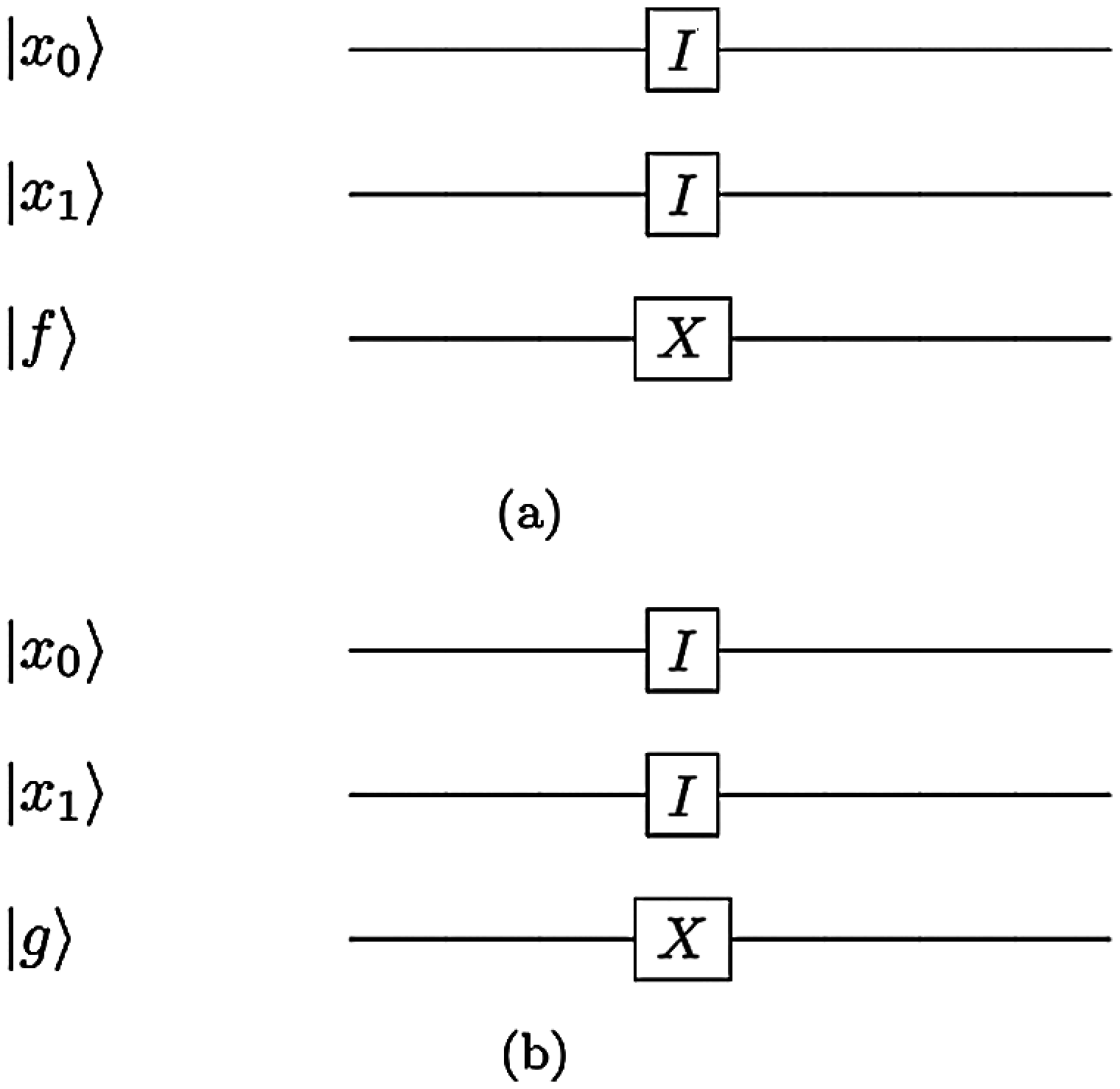Figure 3: The quantum circuits for two different oracles Uf and Ug, respectively, the hamming distance in between is Ham (f(x0, x1), g(x0, x1)) = 0: (a) The quantum circuit of the oracle Uf that represents the Boolean function f(x0, x1) = 1. (b) The quantum circuit of the oracle Ug that represents the Boolean function g(x0, x1) = 1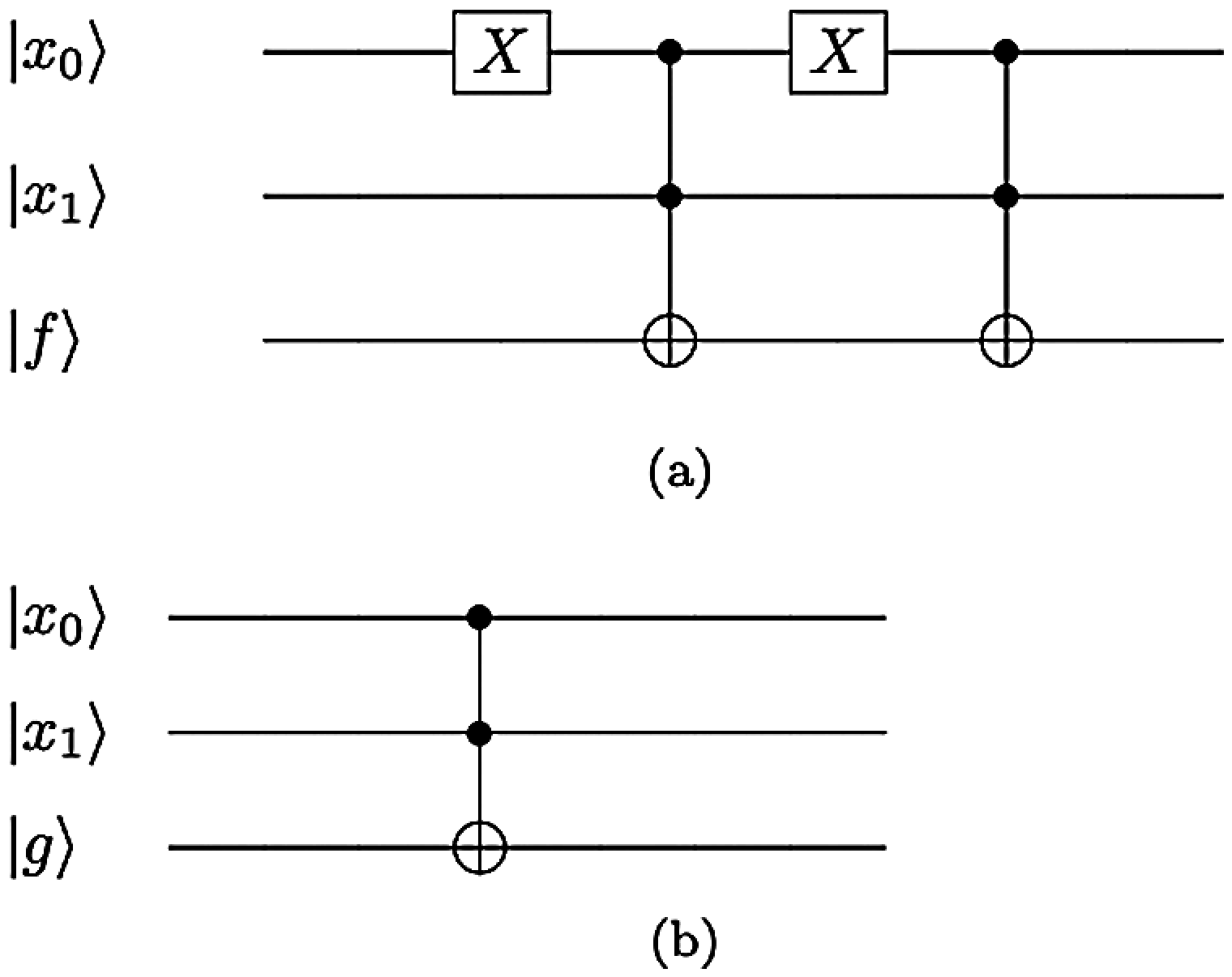Figure 4: The quantum circuits for two different oracles Uf and Ug, respectively. The hamming distance in between is Ham(f(x0, x1), g(x0, x1)) = 1: (a) The quantum circuit of the oracle Uf that represents the Boolean function f(x0, x1) = x1. (b) The quantum circuit of the oracle Ug that represents the Boolean function g(x0, x1) = x0x1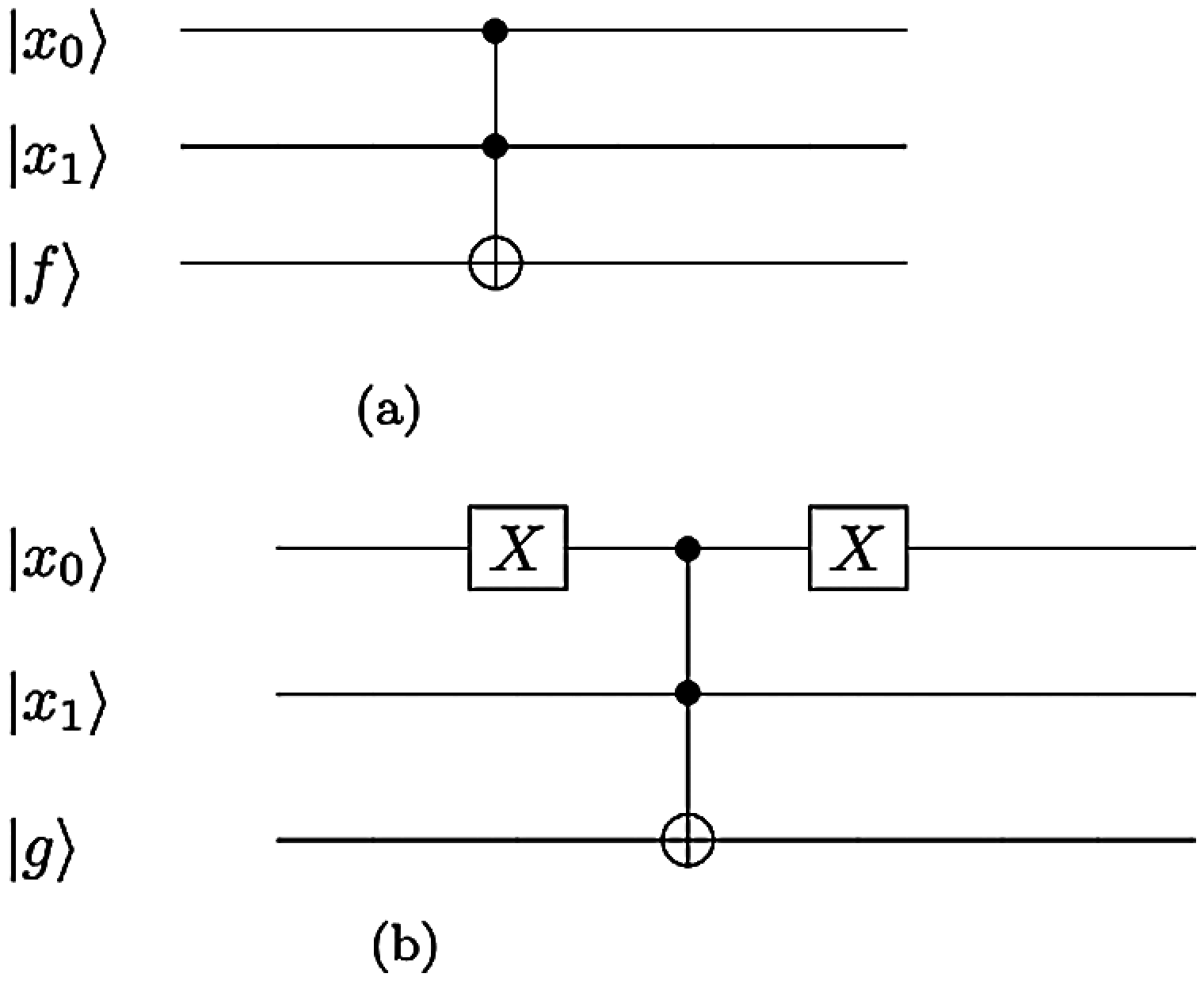Figure 5: The quantum circuits for two different oracles Uf and Ug, respectively. The hamming distance in between is Ham(f(x0, x1), g(x0, x1)) = 2: (a) The quantum circuit of the oracle Uf that represents the Boolean function f(x0, x1) = x0x1. (b) The quantum circuit of the oracle Ug that represents the Boolean function g(x0, x1) = x0x1x1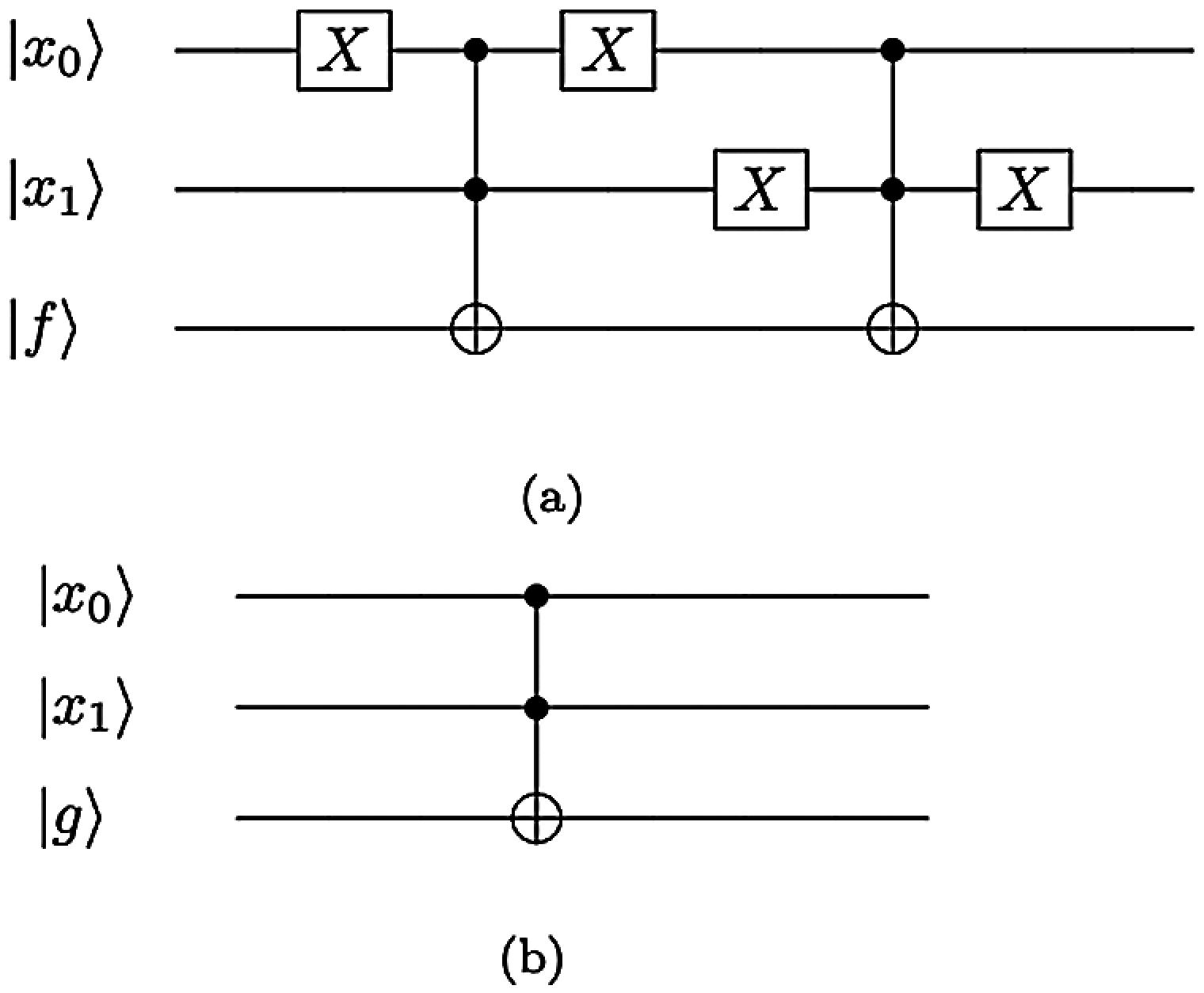Figure 6: The quantum circuits for two different oracles Uf and Ug, respectively. The hamming distance in between is Ham(f(x0, x1), g(x0, x1)) = 3: (a) The quantum circuit of the oracle Uf that represents the Boolean function f(x0, x1) = x0x1. (b) The quantum circuit of the oracle Ug that represents the Boolean function g(x0, x1) = x0x1

4.2 Results Discussion

It is clear from Section 3.2 that the proposed algorithm measures Ham(f(x), g(x)) between two given oracles Uf and Ug based on the concurrence value C by measuring the last four qubits of the system described by Eq. (16). The outcomes of this measurement process estimate the probabilities P0000, P0011, P1100, and P1111 after M shots of measurement. Then, C is calculated quantum mechanically using Eq. (4). The estimated probabilities P0000, P0011, P1100, and P1111 for the five conducted experiments are depicted in Figs. 8a8e. The blue histograms represent the estimation of these probabilities by IBM's simulator and the green histograms represent the theoretical estimation. The results of the first simulation experiment that measures Ham(f(x), g(x)) between the Boolean functions f(x0, x1) = 1 and g(x0, x1) = 1 are shown in Fig. 8a. It shows that both the simulation results of estimating the probabilities P0000, P0011, P1100, and P1111 match the theoretical values exactly with fidelity F = 1. Theoretically, the Hamming distance between the Boolean functions f(x0, x1) = 1, and g(x0, x1) = 1 from their truth tables is Ham(f(x), g(x)) = 0. Practically, it is clear from Fig. 8a that the probabilities P0011, P1100 are zero; this implies that C = 0 from Eq. (4). According to Fig. 8a, it is obvious that P0000 > P1111.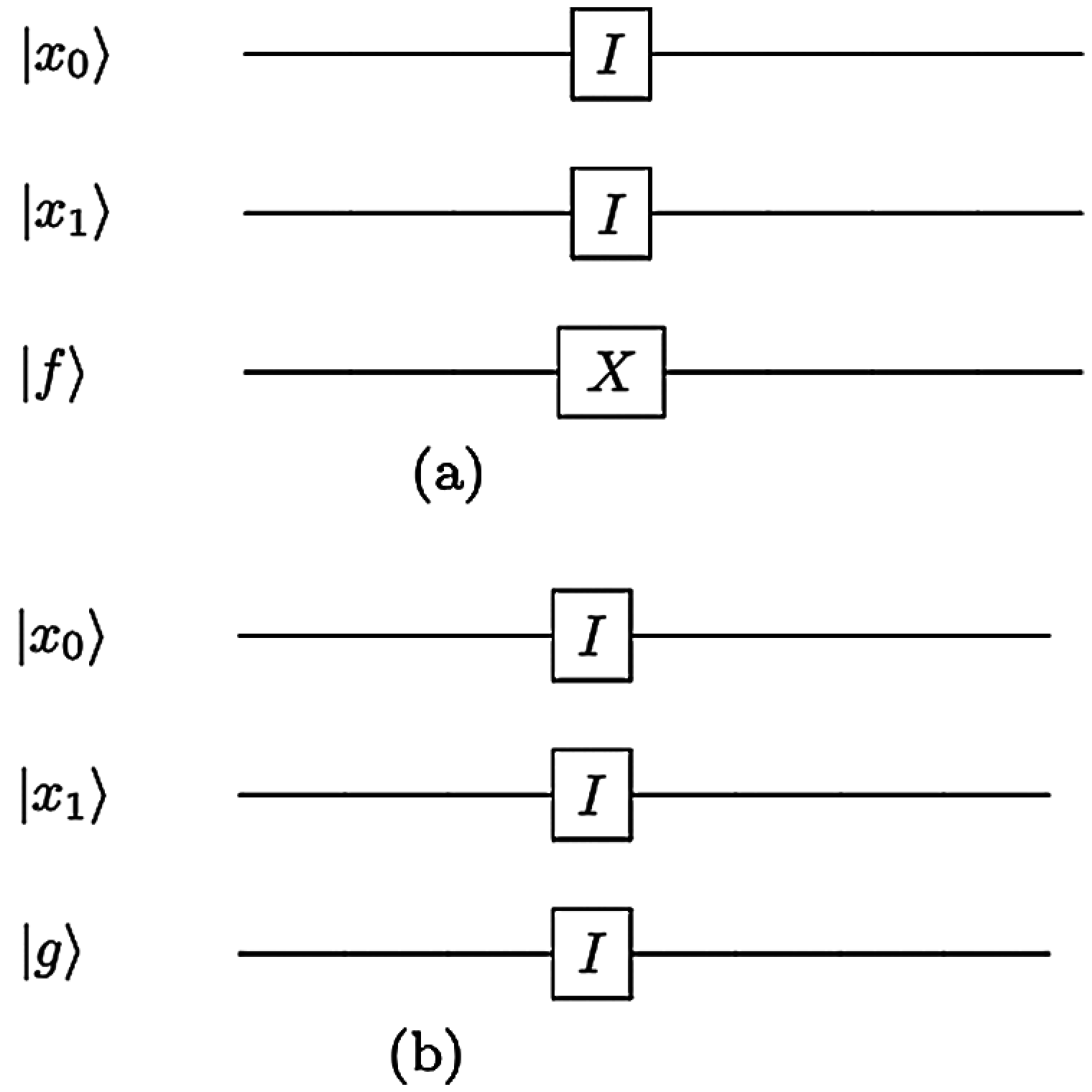Figure 7: The quantum circuits for two different oracles Uf and Ug, respectively. The hamming distance in between is Ham(f(x0, x1), g(x0, x1)) = 4: (a) The quantum circuit of the oracle Uf that represents the Boolean function f(x0, x1) = 1. (b) The quantum circuit of the oracle Ug that represents the Boolean function g(x0, x1) = 0. (a). QHD = 0 (b). QHD = 1 (c). QHD = 2 (d). QHD = 3 (e) QHD = 4

Hence, the simulation result of measuring the hamming distance by the proposed algorithm is Ham(f(x),g(x))=42(110)=0; the same value that is expected theoretically. The results of the second experiment that measures Ham(f(x), g(x)) between the Boolean functions f(x0, x1) = x1, and g(x0, x1) = x0x1 are shown in Fig. 8b. It reveals that both the simulation results of estimating the probabilities P0000, P0011, P1100, and P1111 match the theoretical values expressively with fidelity F =0.999975604. Theoretically, the Hamming distance between the Boolean functions f(x0, x1) = x1, and g(x0, x1) = x0x1 from their truth tables is Ham(f(x), g(x)) = 1. Practically, Fig. 8b shows that the probabilities P0011, P1100 are 0.1912 and 0.1899, respectively. Hence, the concurrence value is C =0.87304469 using Eq. (4). According to Fig. 8b, it is obvious that P0000 >P1111. Therefore, the simulation result of measuring the hamming distance by the proposed algorithm is Ham(f(x),g(x))=42(11(0.87304469)2)=1.0247195921. That matches theoretical result significantly. The results of the third experiment that measures Ham(f(x), g(x)) between the Boolean functions f(x0, x1) = x0x1, and g(x0, x1) = x0x1x1 are shown in Fig. 8c. It indicates that both the simulation results of estimating the probabilities P0000, P0011, P1100, and P1111 match the theoretical values expressively with fidelity F =0.99999766. Theoretically, the Hamming distance between the Boolean functions f(x0, x1) = x0x1, and g(x0, x1) = x0x1x1 from their truth tables is Ham(f(x), g(x)) = 2. Practically, Fig. 8c shows that the probabilities P0011, P1100 are 0.2513 and 0.2487, respectively. Hence, the concurrence value is C =1 by Eq. (4). Fig. 8c manifests that P0000 ≥ P1111. Accordingly, the simulation result of measuring the hamming distance by the proposed algorithm is Ham(f(x),g(x))=42(11(1)2)=2. Hence, in this experiment the theoretical results match the simulation results ideally. The results of the fourth experiment that measures Ham(f(x), g(x)) between the Boolean functions f(x0, x1) = x0x1, and g(x0, x1) = x0x1 are shown in Fig. 8d. It shows that both the simulation results of estimating the probabilities P0000, P0011, P1100, and P1111 match the theoretical values expressively with fidelity F =0.999907003. Theoretically, the Hamming distance between the Boolean functions f(x0, x1) = x0x1, and g(x0, x1) = x0x1 from their truth tables is Ham(f(x), g(x)) = 3.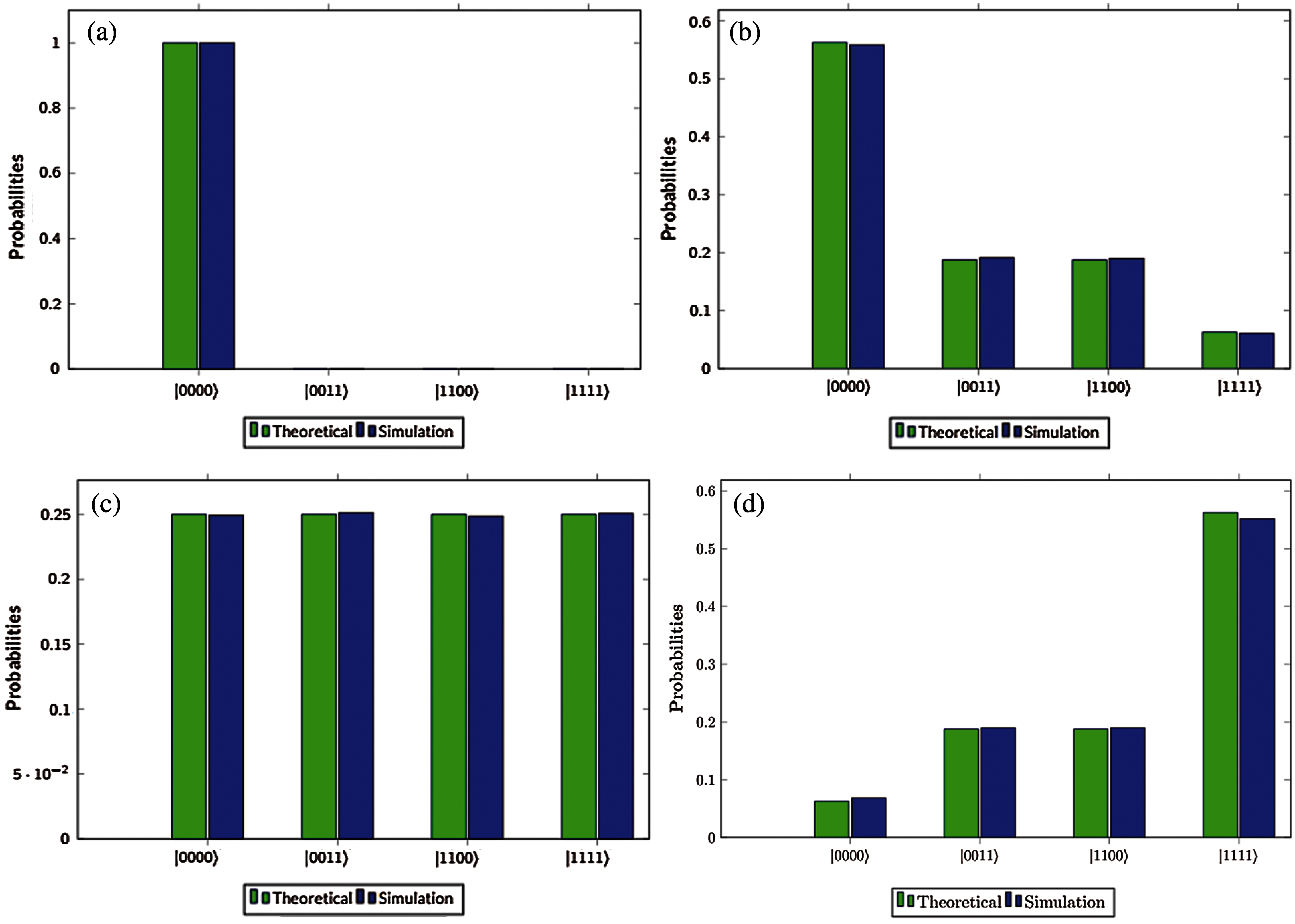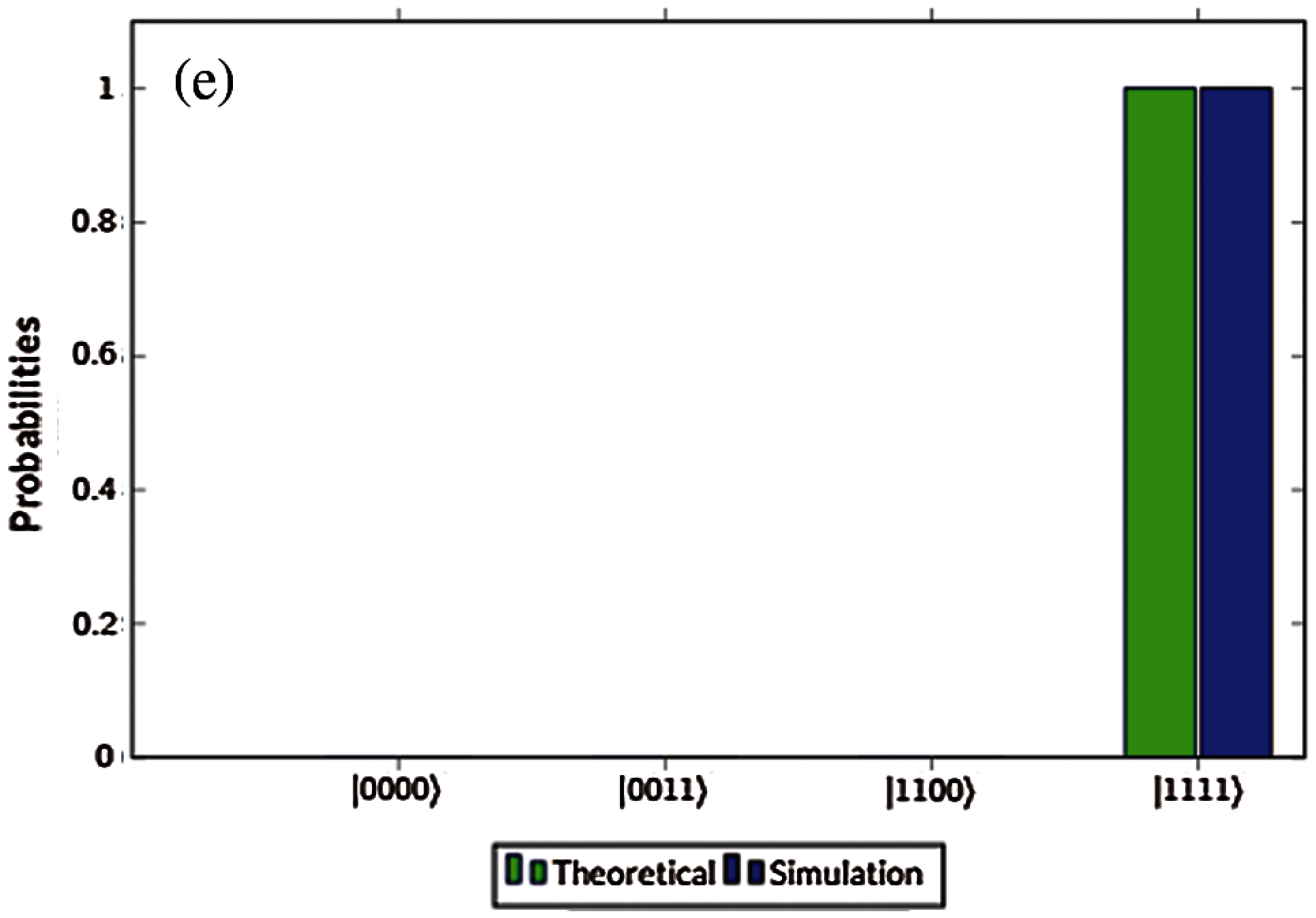Figure 8: Comparison between the theoretical results and simulation results for the proposed algorithm to measure different hamming distance Ham(f(x),g(x)) between two unknown oracles (a) Ham(f(x),g(x))=0; (b) Ham(f(x),g(x))=1; (c) Ham(f(x),g(x))=2; (d) Ham(f(x),g(x))=3; (e) Ham(f(x),g(x))=4

Practically, it is clear from Fig. 8d that P0011 = P1100 = 0.1899, respectively. So, the concurrence value is C = 0.8716 by Eq. (4). Fig. 8d shows that P1111 >P0000. Thus, the simulation result of measuring the hamming distance by the proposed algorithm is Ham(f(x),g(x))=42(11+(0.8716)2)=2.9802741963. Thus, both of the experimental results and the theoretical results are consistent. The results of the last experiment that measures Ham(f(x), g(x)) between the Boolean functions f(x0, x1) = 1 and g(x0, x1) = 0 are shown in Fig. 8e. It shows that both the simulation results of estimating the probabilities P0000, P0011, P1100, and P1111 match the theoretical values exactly with fidelity F =1. Theoretically, the Hamming distance between the Boolean functions f(x0, x1) = 1, and g(x0, x1) = 0 from their truth tables is Ham(f(x), g(x)) = 4. Practically, it is clear from Fig. 8e that the probabilities P0011, P1100 are zero; this implies that C = 0 from Eq. (4).

According to Fig. 8e, P1111 >P0000, so the simulation result of measuring the hamming distance by the proposed algorithm is Ham(f(x),g(x))=42(1+10)=4; the same value that is expected theoretically. Overall, the results of the simulations show that the proposed algorithm is verified with reliable fidelity. The Quantum Hamming Distance (QHD) is the Ham(f(x),g(x)) investigated in Fig. 8 such that 0, 1, 2, 3 and 4 in Figs. 8a8e, respectively.

5  Conclusions

In this work, a novel quantum algorithm that measures the Hamming distance between two given oracles is explained. The proposed algorithm retrieves the hamming distances based on the degree of the entanglement between two auxiliary qubits. Therefore, the analysis of the performance of the proposed algorithm is investigated via a case study. Then, the complexity of the proposed algorithm compared to classical algorithm is explained in detail. Finally, the algorithm is verified by IBM's quantum computer simulator. The simulations results shows that the performance of the proposed algorithm is verified with fidelity close to 1.

Funding Statement: The authors received no specific funding for this study.

Conflicts of Interest: The authors declare that they have no conflicts of interest to report regarding the present study.

References

1.  M. Abdel-Aty, “Delayed sudden birth and sudden death of entanglement in josephson-charge qubits,” Laser Physics, vol. 19, no. 3, pp. 511–515, 2009.

2.  M. Abdel-Aty, J. Larson, H. Eleuch and A. S. Obada, “Multi-particle entanglement of charge qubits coupled to a nanoresonator,” Physica E: Low-Dimensional Systems and Nanostructures, vol. 43, no. 9, pp. 1625–1630, 2011.

3.  A. Drucker and R. De Wolf, “Uniform approximation by (quantum) polynomials,” Arxiv Preprint Arxiv:1008.1599, pp. 1–9, 2010.

4.  A. Nayak and F. Wu, “The quantum query complexity of approximating the median and related statistics,” in Proc. of the Thirty-First Annual ACM Symposium on Theory of Computing, Atlanta, Georgia, USA, pp. 384–393, 1999.

5.  E. Bernstein and U. Vazirani, “Quantum complexity theory,” SIAM Journal on Computing, vol. 26, no. 5, pp. 1411–1473, 1997.

6.  A. De, I. Diakonikolas, V. Feldman and R. A. Servedio, “Nearly optimal solutions for the chow parameters problem and low-weight approximation of halfspaces,” Journal of the ACM (JACM), vol. 61, no. 2, pp. 1–36, 2014.

7.  M. Zidan, “A novel quantum computing model based on entanglement degree,” Modern Physics Letters B, vol. 34, no. 35, pp. 2050401, 2020.

8.  Z. Xie, D. Qiu and G. Cai, “Quantum algorithms on walsh transform and hamming distance for boolean functions,” Quantum Information Processing, vol. 17, no. 6, pp. 1–17, 2018.

9.  K. Kathuria, A. Ratan, M. McConnell and S. Bekiranov, “Implementation of a hamming distance–like genomic quantum classifier using inner products on ibmqx2 and ibmq_16_melbourne,” Quantum Machine Intelligence, vol. 2, no. 1, pp. 1–26, 2020.

10. J. F. Doriguello and A. Montanaro, “Quantum sketching protocols for hamming distance and beyond,” Physical Review A, vol. 99, no. 6, pp. 062331, 2019.

11. M. Zidan, A. H. Abdel-Aty, D. M. Nguyen, A. S. Mohamed, Y. Al-Sbou et al., “A quantum algorithm based on entanglement measure for classifying boolean multivariate function into novel hidden classes,” Results in Physics, vol. 15, pp. 102549, 2019.

12. A. H. Abdel-Aty, H. Kadry, M. Zidan, Y. Al-Sbou, E. A. Zanaty et al., “A quantum classification algorithm for classification incomplete patterns based on entanglement measure,” Journal of Intelligent & Fuzzy Systems, vol. 38, no. 3, pp. 2809–2816, 2020.

13. M. Zidan, H. Eleuch and M. Abdel-Aty, “Non-classical computing problems: Toward novel type of quantum computing problems,” Results in Physics, vol. 21, pp. 103536, 2021.

14. D. M. Nguyen and S. Kim, “New construction of binary and nonbinary quantum stabilizer codes based on symmetric matrices,” International Journal of Modern Physics B, vol. 33, no. 24, pp. 1950274, 2019.

15. D. M. Nguyen and S. Kim, “A novel construction for quantum stabilizer codes based on binary formalism,” International Journal of Modern Physics B, vol. 34, no. 8, pp. 2050059, 2020.

16. IBM Quantum Experience, 2020. [Online]. Available: https://www.ibm.com/quantum-computing/.This work is licensed under a Creative Commons Attribution 4.0 International License, which permits unrestricted use, distribution, and reproduction in any medium, provided the original work is properly cited.